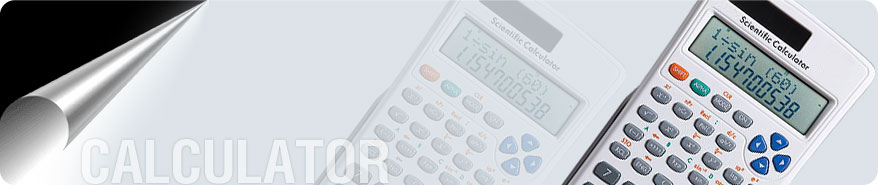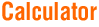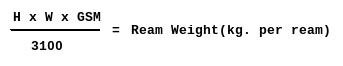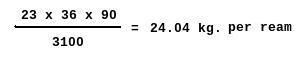Home  |  history | About Us  |  Products  |  Paper Calculator  |  Track n Trace  |  Enquiry Form   |  contact Us· Weight Chart · Caliper Chart · Conversion Chart · Formulas
Calculations & Conversions
How to Determine Ream Weights

To determine the ream weight of any given size sheet, multiply the square inches in the given size by the given basis weight; divide the result by the square inch area of the basic size

 Online Calculation Given Size (height): Given Size (width): Grammage: Ream Weight: kg. per ream

 FormulaExample Find the ream weight of 23 X 36 (in) 90 gsm per ream.